www.batmath.it

### Fundamental theorem of the integral calculus

The integral function F and the integrand function f give an example of two functions with F'=f. In general we give the following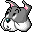Definition

If f is a function whose domain is an interval I of real numbers, we call antiderivative of f every function F such that, for all x in I, the following property holds: F'=f.

For example sinx is an antiderivative of cosx, as (sinx)'=cosx.

Observe that F is an antiderivative if the property F'=f  holds for every x in I. So antiderivative is a set property, and it makes no sense to speak of antiderivative in a point.

In this site ou can find a complete study of the concept of antiderivative (in Italian). Here we only remind that a special symbol is used to represent the set of all antiderivatives of a given function f: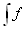or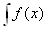or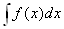, that is almost the same symbol used for the Riemann integral, without the bounds. But pay attention: these symbols represent a set of functions, not a real number!

#### Continuity of the integral function

The integral function of a given function f is always more regular than f. The following theorem concerns its continuity.Theorem

The integral function of a Riemann integrable function is always continuous.Let's consider an example using the integral part function (this is a step function, so its integral is very simple). It is not difficult to prove that, in the interval [-3,3[, we have: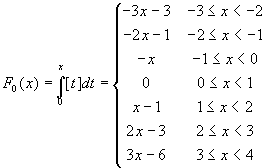. In order to do this observe that, for positive x you must calculate the yellow area in the picture below on the left, for negative x the green area. In the picture below, on the right we have represented this integral function.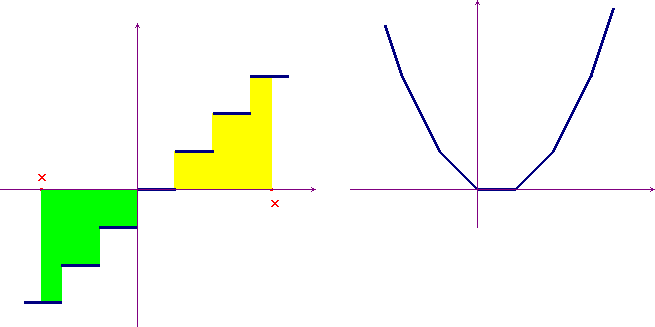#### The fundamental theorem of integral calculus

The following theorem, as its name shows, is one of the most important outcomes of analysis. It allows us to calculate, by a very simple technique, a wide class of integrals, without using a direct calculation based on the definition.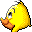Theorem

If an integrable functionis continuous in a point d, then the integral functions, with starting point each number c of [a,b], are differentiable in d, and Fc'(d)=f(d).The main consequence of this theorem is that if a function is continuous in an interval [a,b], the integral functions with starting points all points in [a,b] are antiderivatives of f (however in this way we do not obtain all the antiderivatives of f, as we'll see): so continuous functions have always antiderivatives.

This theorem is known as the Torricelli-Barrow theorem.

#### Examples

• The function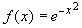is continuous everywhere. As a consequence the functions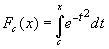are differentiable functions, and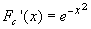, so they are also monotòne increasing functions. These functions are not elementary functions: there is no way to represent them by means of a finite number of sums, multiplications, divisions on the independent variable, nor by means of functions such as exponentials, logarithms, trigonometric functions. The proof of this fact, however, is not easy at all, and is far beyond the limits of this presentation of the Riemann integral.
• The same considerations apply to the function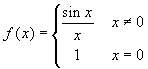whose integral functions are not elementary functions. However they are differentiable and their derivative is always f(x).
• Considering again the function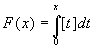, its easy to prove that it is differentiable everywhere, except at the points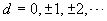, where the function [x] is not continuous. At all points where the function is differentiable the equality F'(x)=[x] holds.

#### Calculation of integrals

We'll now show how, using the fundamental theorem, one can easily calculate Riemann integrals, provided an antiderivative is known.

Let G(x) be an antiderivative of a continuous function, then as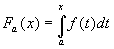is also an antiderivative, we must have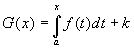. But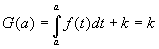and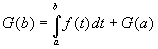. We may conclude that. This equality is usually written as.

This result is summarized by the following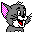Theorem

If G(x) is an antiderivative of a continuous function, then.

This theorem is sometimes called The second fundamental theorem of integral calculus: if you know an antiderivative of a continuous function, the calculation of the Riemann integral is only a matter of substitution!

first published on january 07 2003 - last updated on september 01 2003